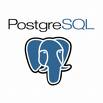Last week, someone asked me what a "perfect" dashboard for a network would look like. Trying to channel Tufte, I attempted to list all the variables that the viewer would care about. Since management uses a dashboard more often than other users, that'd be my audience. I reduced everything down to two variables: time and percentage of customers affected. Easy enough, right?

However, management probably wants to see the recent "in focus" and see how that flows into the long run (comparing the detailed now to the full history of the enterprise). That potentially large scale rules out a simple linear domain for the chart, and implies that a logarithmic domain would work better. something like the following chart (not pretty enough for a dashboard, but you get the idea;):

So let's create a postgresql function to transform numeric input into logarithmic buckets for grouping easily and efficiently:

create or replace function logarithmic_axis(numeric, numeric, numeric, numeric) returns numeric as \$\$ /* return a logarithmic chunked display of data from table XXX: with small width's, the time delta between periodic sampling dominates */ declare stamp alias for \$1; -- current epochal time to bucketize init alias for \$2; -- beginning of time range fin alias for \$3; -- end of time range width alias for \$4; -- how many buckets do we want delta numeric = 0.0; rate numeric = 0.0; exp numeric = 0.0; begin delta := fin - init; rate := pow(delta, (1 / width) ); delta := fin - stamp; if delta = 0 then -- catch the last row delta := 1; end if; exp := log(rate, cast(delta as numeric) ); exp := floor(exp); return (fin - floor( pow(rate, exp) ) ); end; \$\$ LANGUAGE plpgsql;

An example usage of this goes like (and is what generated the chart above):

select to_timestamp(logarithmic_axis( cast(extract(epoch from stocks.day) as numeric), (select cast(extract(epoch from min(day)) as numeric) from stocks), (select cast(extract(epoch from max(day)) as numeric) from stocks), 120)) as l, avg(price) from stocks group by l order by l;